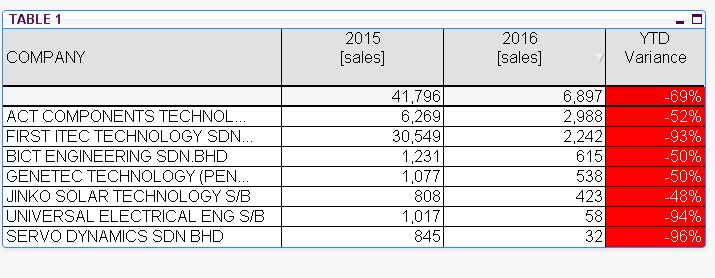# App Development

Announcements
Customer & Partners, DEC. 9, 11 AM ET: Qlik Product & Strategy Roadmap Session: Data Analytics REGISTER NOW
cancel
Showing results for
Did you mean:Master

## Why i insert sale={'0'} into my SET expression , it unable filter off those row with zero ?

TABLE 1 EXPRESSION IS OKAY :-

if(

Sum({\$<year = {\$(=Max(year)-1)}, month = {"<=\$(=Max({<year={\$(=Max(year))}, sales = {'*'}>} month))"}>}sales/Rate)

>=

Sum({\$<year = {\$(=Max(year)-0)}, month = {"<=\$(=Max({<year={\$(=Max(year))}, sales = {'*'}>} month))"}>}sales/Rate)

,

Sum({\$<year = {\$(=Max(year)-0)}, month = {"<=\$(=Max({<year={\$(=Max(year))}, sales = {'*'}>} month))"}>}sales/Rate)

)

IN ORDER TO FILTER OFF ZERO ROW , I ADD sale={'0'} TO ABOVE EXPRESSION :-

if(

Sum({\$<year = {\$(=Max(year)-1)}, month = {"<=\$(=Max({<year={\$(=Max(year))}, sales = {'*'},sale={'0'}>} month))"}>}sales/Rate)

>=

Sum({\$<year = {\$(=Max(year)-0)}, month = {"<=\$(=Max({<year={\$(=Max(year))}, sales = {'*'},sale={'0'}>} month))"}>}sales/Rate)

,

Sum({\$<year = {\$(=Max(year)-0)}, month = {"<=\$(=Max({<year={\$(=Max(year))}, sales = {'*'},sale={'0'}>} month))"}>}sales/Rate)

)

But it never work. See Table 2., Now it display total 18 company , i need it display only 1 to 3 row.

1 Solution

Accepted SolutionsMVP

Change your first expression as below

IF(Column(2), if(

Sum({\$<year = {\$(=Max(year)-0)}, month = {"<=\$(=Max({<year={\$(=Max(year))}, sales = {'*'}>} month))"}>}sales/Rate)

<=

Sum({\$<year = {\$(=Max(year)-1)}, month = {"<=\$(=Max({<year={\$(=Max(year))}, sales = {'*'}>} month))"}>}sales/Rate)

,

Sum({\$<year = {\$(=Max(year)-1)}, month = {"<=\$(=Max({<year={\$(=Max(year))}, sales = {'*'}>} month))"}>}sales/Rate)

))

You will get result like below..5 RepliesMaster
Author

my QVWMVP

Change your first expression as below

IF(Column(2), if(

Sum({\$<year = {\$(=Max(year)-0)}, month = {"<=\$(=Max({<year={\$(=Max(year))}, sales = {'*'}>} month))"}>}sales/Rate)

<=

Sum({\$<year = {\$(=Max(year)-1)}, month = {"<=\$(=Max({<year={\$(=Max(year))}, sales = {'*'}>} month))"}>}sales/Rate)

,

Sum({\$<year = {\$(=Max(year)-1)}, month = {"<=\$(=Max({<year={\$(=Max(year))}, sales = {'*'}>} month))"}>}sales/Rate)

))

You will get result like below..Master
Author

Hi Manish

Very smart move. Thank you.

PaulMaster
Author

Hi Sir

Can you share with me what is the meaning for below script ?

IF(Column(2), if(

As i ready don't know where to start to modify your script , so that i can list row 8 till 27 , as i also need the list of customer stop buiuying .

PaulMVP

If(Column(2) means 2nd expression... if value is other than 0 then only 1st expression will give you value otherwise null.Tags
Community Browser